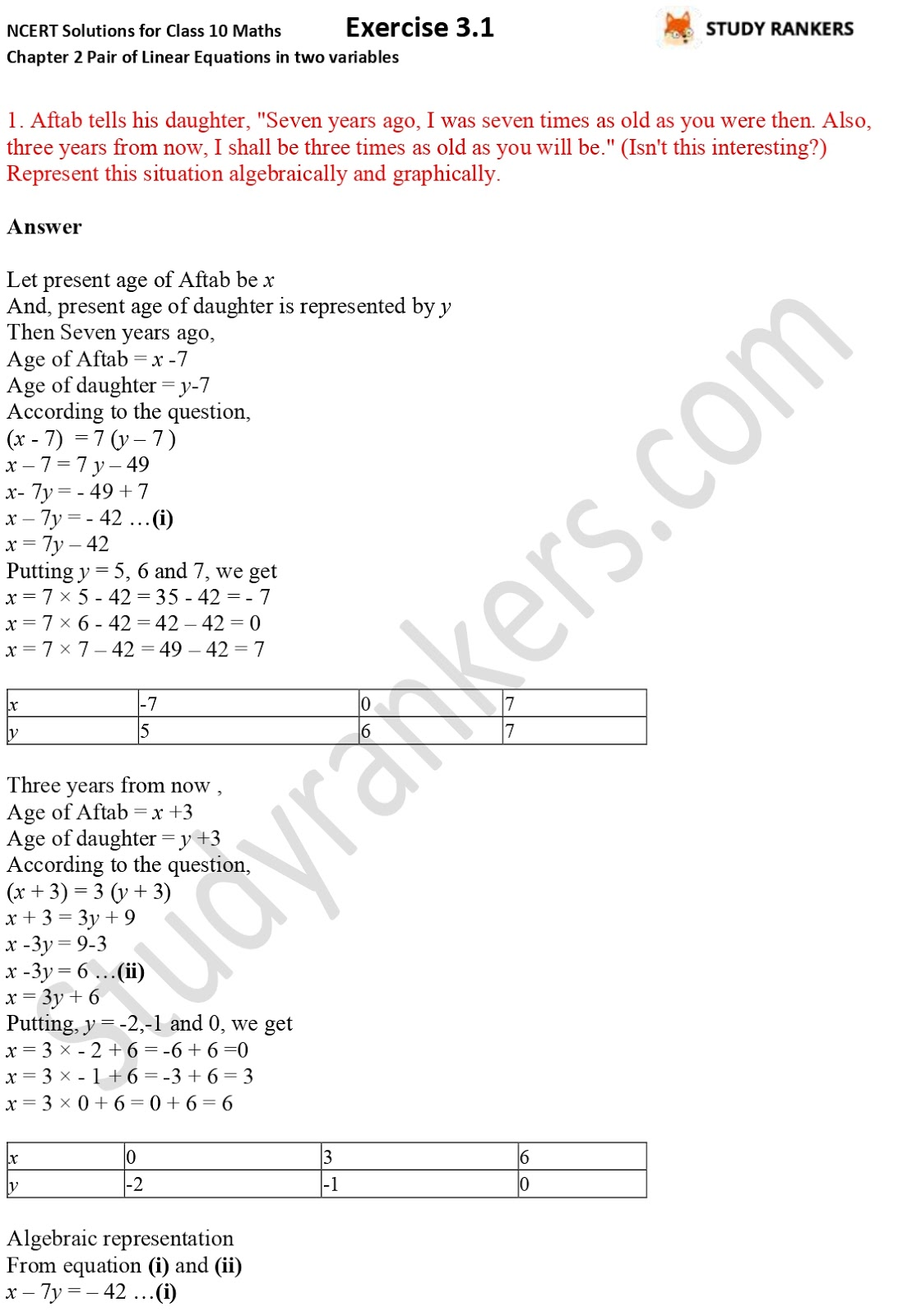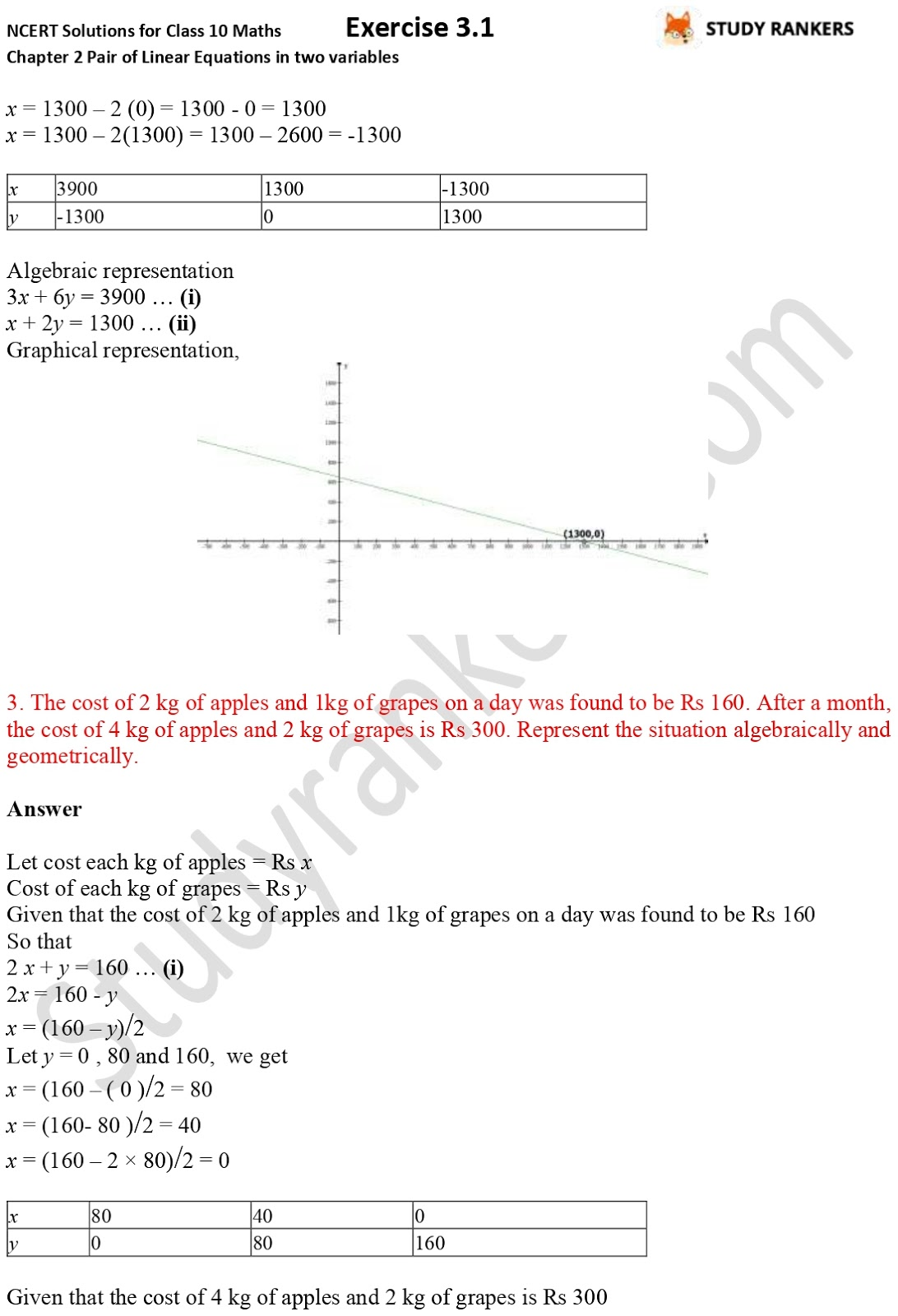>

## NCERT Solutions for Class 10 Maths Chapter 3 Pair of Linear Equations in Two Variables Exercise 3.1

If you are in need of Chapter 3 Exercise 3.1 NCERT Solutions Class 10 Maths then you're at right place. Here we have provided accurate and detailed CBSE NCERT Solutions that can fetch you good marks in the Class 10 board examinations. You can complete homework and understand concepts by solving the exercise and practicing them. The exercise has total three questions, all of them are word problems where you have to represent the situation algebraically and graphically.X